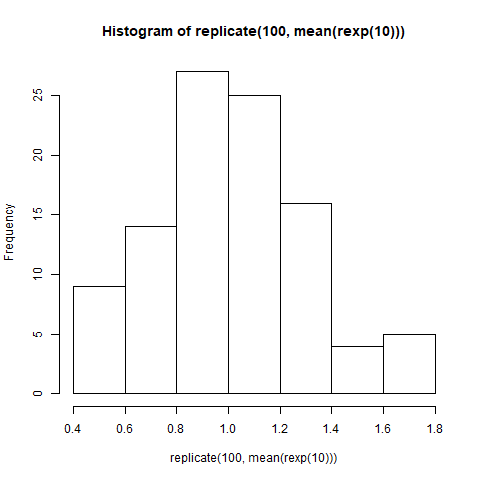Open in App
Not now

# Repeatedly Evaluate an Expression in R Programming – replicate() Function

• Last Updated : 30 Jun, 2020

`replicate()` function in R Language is used to repeatedly evaluate a function or expression. It is member of `apply` family in R base package. In this article, we’ll learn syntax and implementation of `replicate()` function with the help of examples.

Syntax: replicate(n, expr, simplify)

Parameters:
n: represents number of times the expression has to be evaluated
expr: represents expression
simplify: represents logical value. If TRUE, output is represented in vector or matrix form, otherwise in a list form

Example 1:

 `# Set the seed ` `set.seed``(10) ` ` `  `# Generate random numbers with mean = 0 and sd = 1 ` `x <- ``rnorm``(5, mean = 0, sd = 1) ` ` `  `# Print ` `print``(x) ` ` `  `# Evaluating it repeatedly ` `r <- ``replicate``(n = 3, ``rnorm``(5, 0, 1), simplify = ``FALSE` `) ` ` `  `# Print ` `print``(r) `

Output:

```  0.01874617 -0.18425254 -1.37133055 -0.59916772  0.29454513

[]
  0.3897943 -1.2080762 -0.3636760 -1.6266727 -0.2564784

[]
  1.1017795  0.7557815 -0.2382336  0.9874447  0.7413901

[]
  0.08934727 -0.95494386 -0.19515038  0.92552126  0.48297852
```

Example 2:

 `# Output to be present as PNG file ` `png``(file = ``"replicateGFG.png"``) ` ` `  `# Set the seed ` `set.seed``(10) ` ` `  `# Replicate values and create histogram ` `hist``(``replicate``(100, ``mean``(``rexp``(10)))) ` ` `  `# Saving the file ` `dev.off``() `

Output:My Personal Notes arrow_drop_up
Related Articles